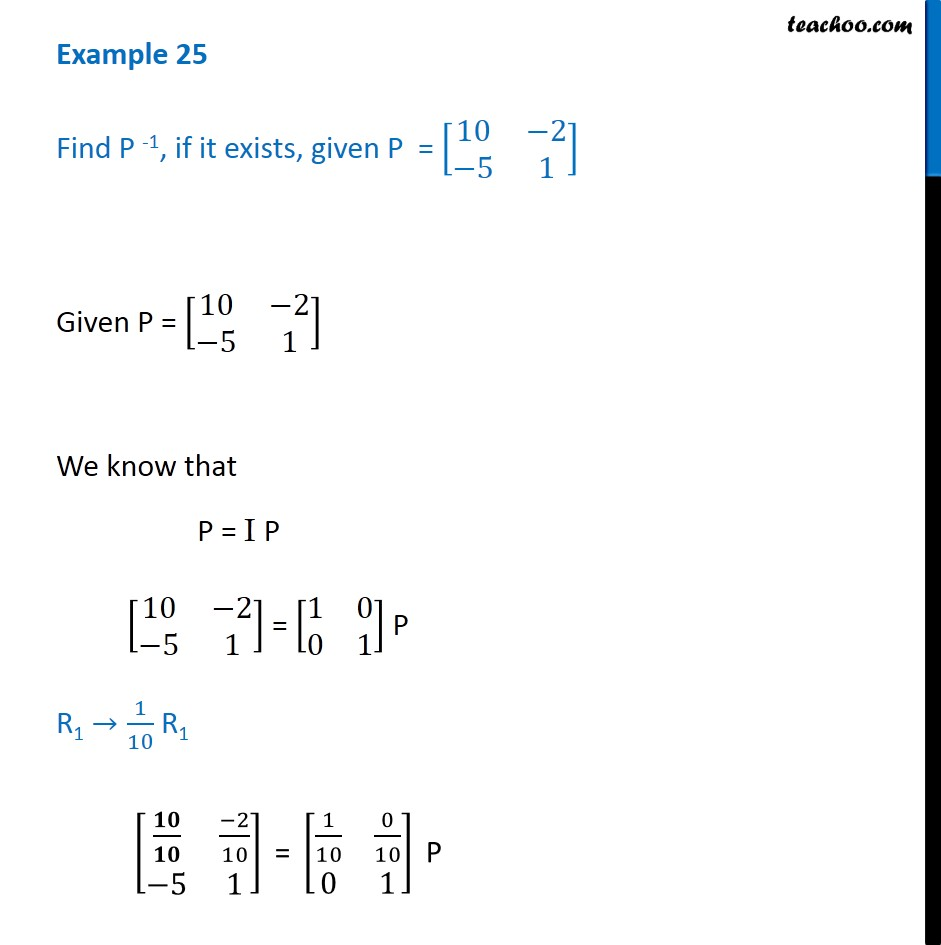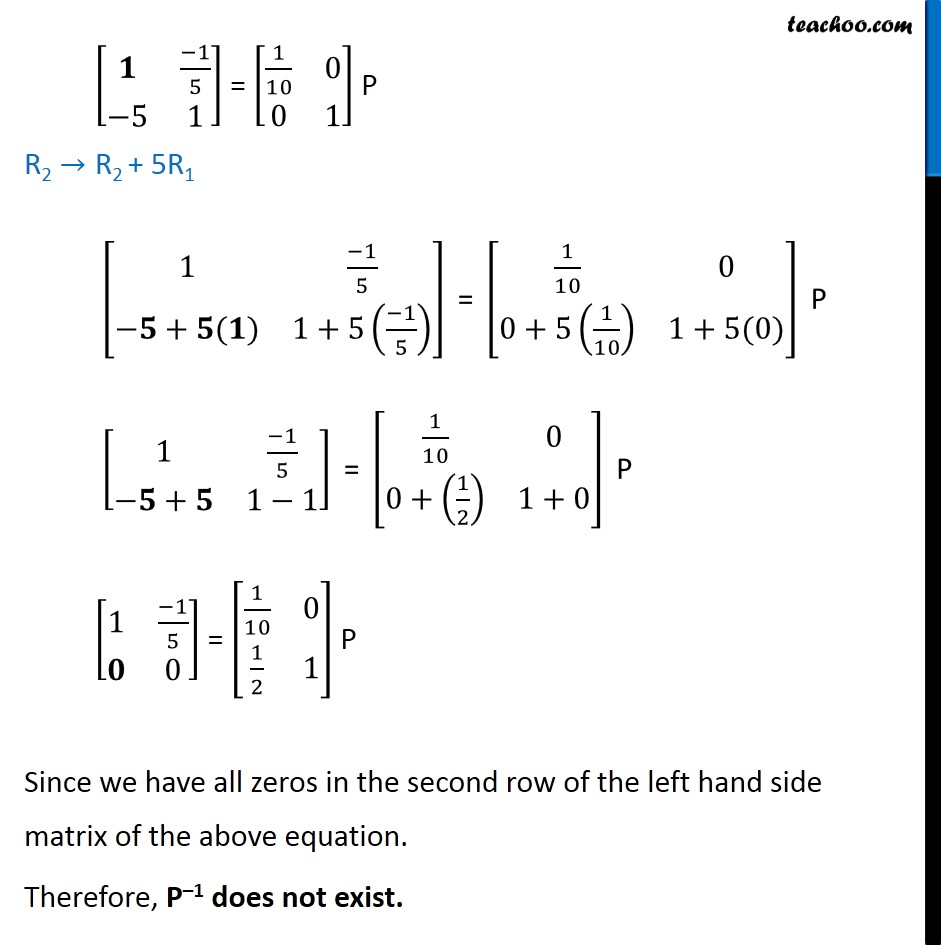Examples

Chapter 3 Class 12 Matrices
Serial order wiseLearn in your speed, with individual attention - Teachoo Maths 1-on-1 Class

### Transcript

Question 3 Find P -1, if it exists, given P = [■8(10&−2@−5&1)] Given P = [■8(10&−2@−5&1)] We know that P = I P [■8(10&−2@−5&1)] = [■8(1&0@0&1)] P R1 →1/10 R1 [■8(𝟏𝟎/𝟏𝟎&(−2)/10@−5&1)]" = " [■8(1/10&0/10@0&1)]" P" [■8(𝟏&(−1)/5@−5&1)] = [■8(1/10&0@0&1)] P R2 →"R2" + 5R1 [■8(1&(−1)/5@−𝟓+𝟓(𝟏)&1+5((−1)/5) )]" = " [■8(1/10&0@0+5(1/10)&1+5(0))]" P" [■8(1&(−1)/5@−𝟓+𝟓&1−1)]" = " [■8(1/10&0@0+(1/2)&1+0)]" P" [■8(1&(−1)/5@𝟎&0)] = [■8(1/10&0@1/2&1)] P Since we have all zeros in the second row of the left hand side matrix of the above equation. Therefore, P–1 does not exist.